# How to generate all combinations of 3 or multiple columns in Excel?

This article describes the user with 3 possible ways to generate the possible combination of 3 or more available columns in Excel. The first example is based on using the user-defined formula, to generate the possible combination. The second example is based on the method to use the VBA code, to generate the required combination. While the last example is based on using the Kutool to generate the same data. All three methods are completely accurate and can generate valid and unique results.

## Example 1: To generate the combination of 3 or multiple columns in Excel by using the formula

### Step 1

To understand the process to generate the combination of 3 or multiple rows, user need to consider below given data −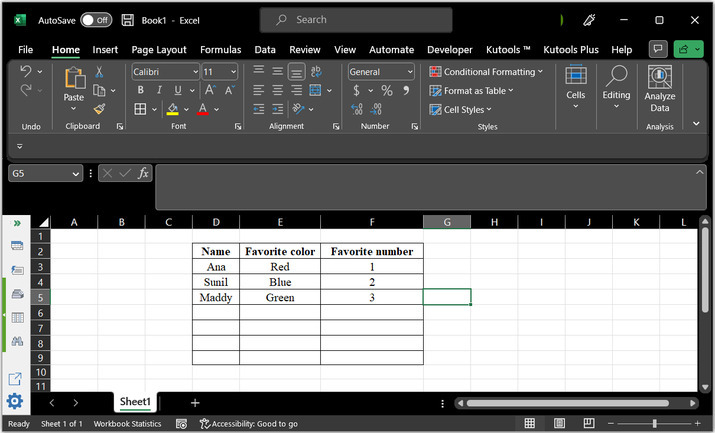### Step 2

Go to the H2 cell and type the below provided formula to the cell −

=IFERROR(INDEX($D$2:$D$4,INT((ROW(1:1)-1)/((COUNTA($E$2:$E$4)*(COUNTA($F$2:$F$4)))))+1)&"-"&INDEX($E$2:$E$4,MOD(INT((ROW(1:1)-1)/
COUNTA($F$2:$F$4)),COUNTA($E$2:$E$4))+1)&"-"&INDEX($F$2:$F$4,MOD((ROW(1:1)-1),COUNTA($F$2:$F$4))+1),"")


Consider below provided image for reference −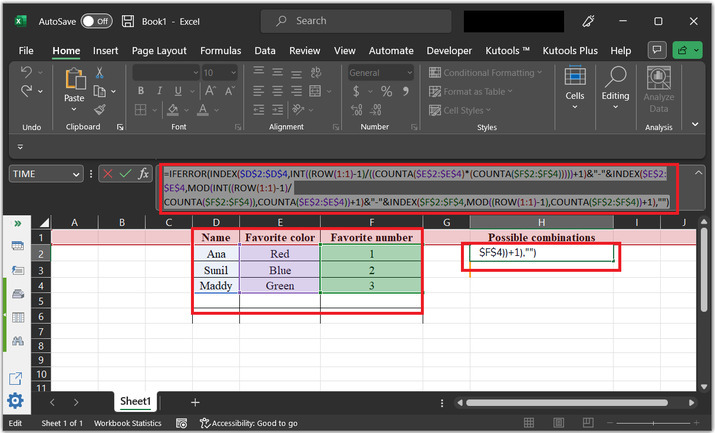### Step 3

Press “Enter” key. After that drag the “+” sign to the bottom of the row. This will generate and type the possible combinations.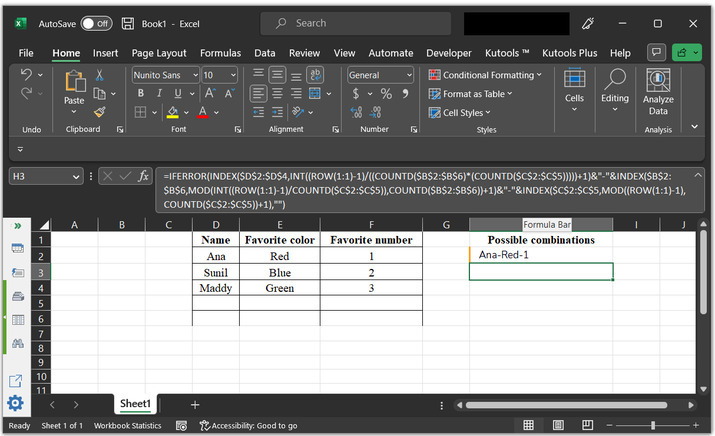### Step 4

Final obtained output, snapshot is provided below −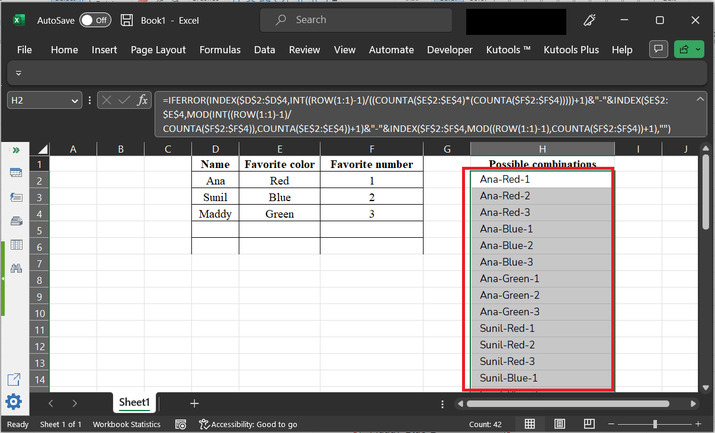## Example 2: To generate the combination of 3 or multiple columns in excel by using the VBA code

### Step 1

In this example, will observe the way to generate the possible combinations, by using the VBA code. After that go to the “Developer” tab and then choose the option that is “Visual Basic” as highlighted in the below image.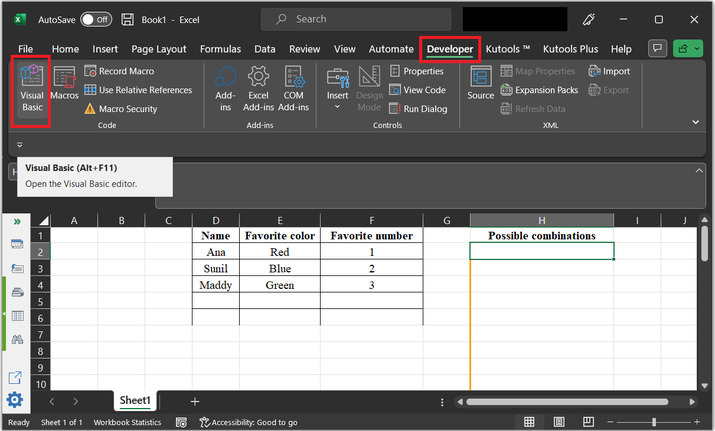### Step 2

This will open the “Microsoft Visual Basics for Applications” dialog box, as shown below −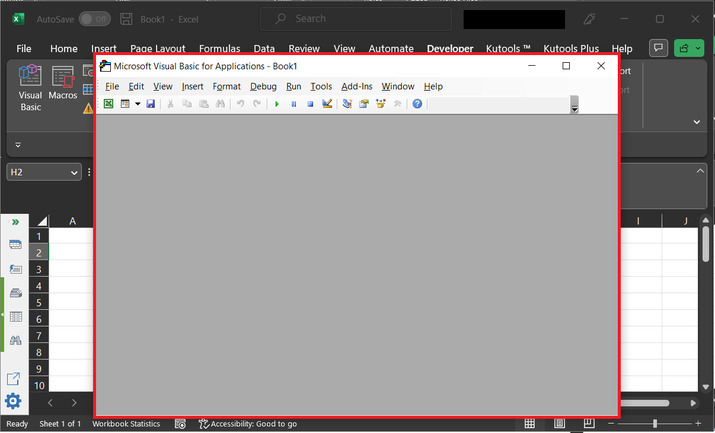### Step 3

After that go to the “Insert” module, and then choose the option “Module”. Consider the below-given snapshot for reference −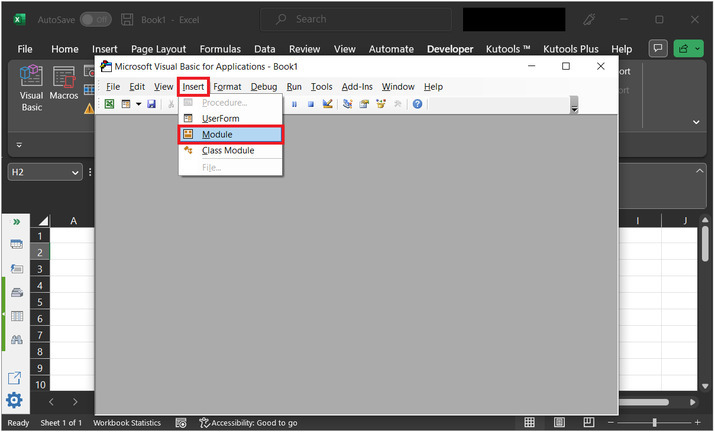### Step 4

This will open a code area. Go to the code area, and type the below-given code −

'Define function header
Sub Combination_List()
' declare required variables for range
Dim x1, x2, x3 As Range
Dim x_range  As Range
' declare a string variable
Dim str_x As String
' declare Integer variable
Dim x1_f, x2_f, x3_f As Integer
Dim x1_s, x2_s, x3_s As String
Set x1 = Range("D2:D4")
Set x2 = Range("E2:E4")
Set x3 = Range("F2:F4")
' setting Separator
str_x = "-"   'Separator
' setting output range
' Output cell
Set x_range = Range("H2")
' for each loop
For x1_f = 1 To x1.Count
x1_s = x1.Item(x1_f).Text
' nested for loop expressions
For x2_f = 1 To x2.Count
x2_s = x2.Item(x2_f).Text
For x3_f = 1 To x3.Count
x3_s = x3.Item(x3_f).Text
x_range.Value = x1_s & str_x & x2_s & str_x & x3_s
Set x_range = x_range.Offset(1, 0)
Next
Next
Next
' end of module
End Sub


Please note that VBA should only run when written properly. This simply mean that the syntax and provided commands must be valid to execute the code.

Consider the code snapshots for proper reference −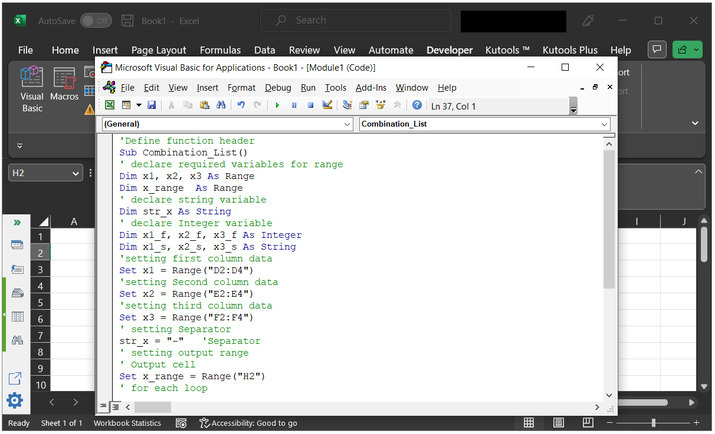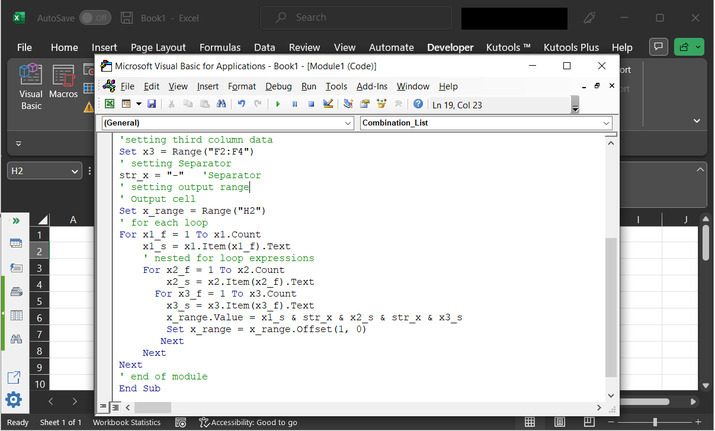### Step 5

After that press “Ctrl + s” to save the data. Go back to the sheet, and user will obtain the below provided output.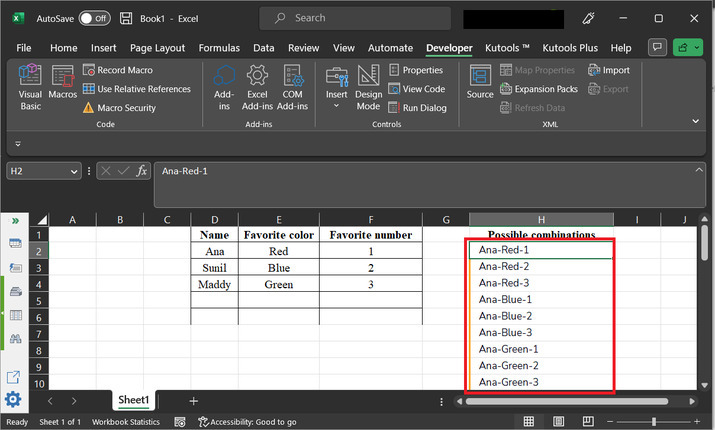## Example 3: To generate the combination of 3 or multiple columns in excel by using the Kutools

### Step 1

This example uses the kutool, to achieve the required task. Consider the same worksheet with initial state, and after that go to the “Kutools” tab → “Editing” → “Insert” → “List All Combinations”.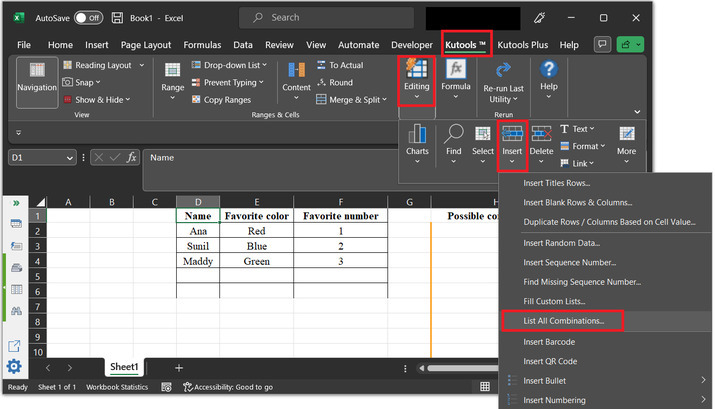### Step 2

This will open the “List All Combinations” dialog box. Consider below given snapshot for proper reference −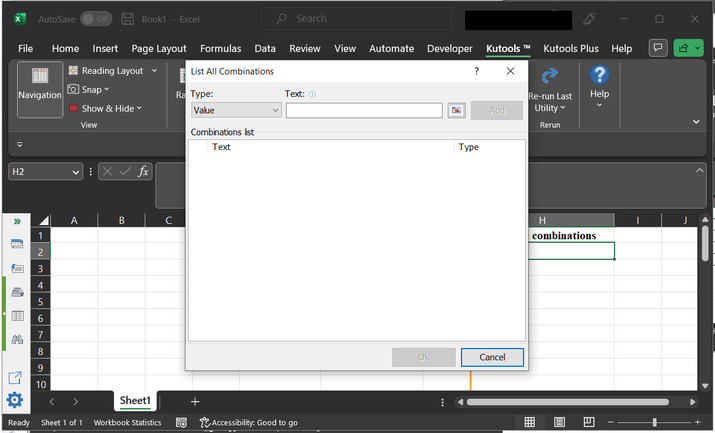### Step 3

Select the below highlighted button, to select the data range one by one.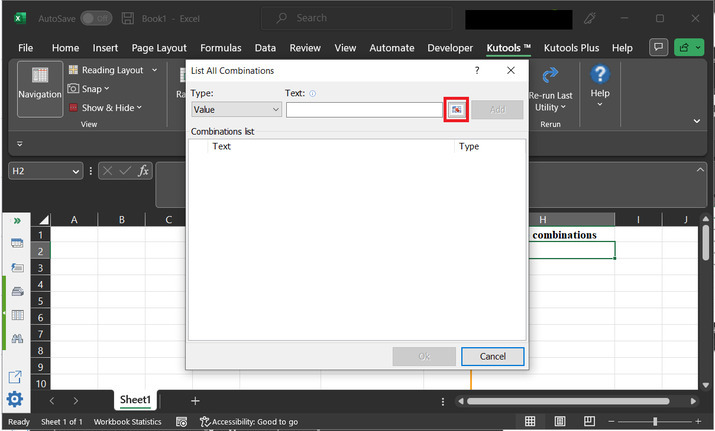### Step 4

First, select the data range from the D column, and click on “OK”.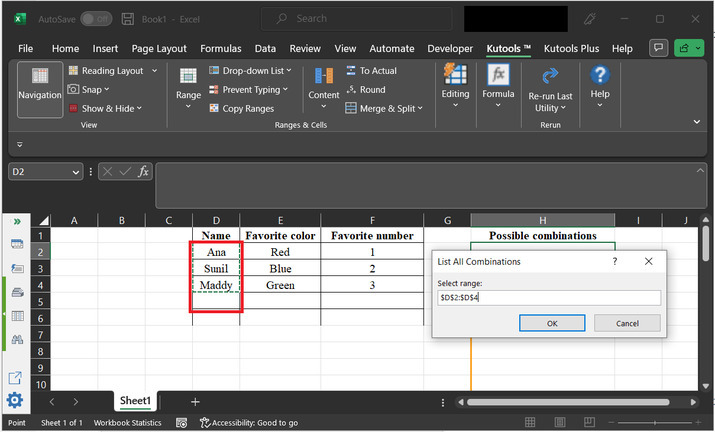### Step 5

Coming back to the “List All Combinations” dialog box, and click on the “Separator” option under the “Type”, and choose “-” in the “Text” section, and click on “Add” button.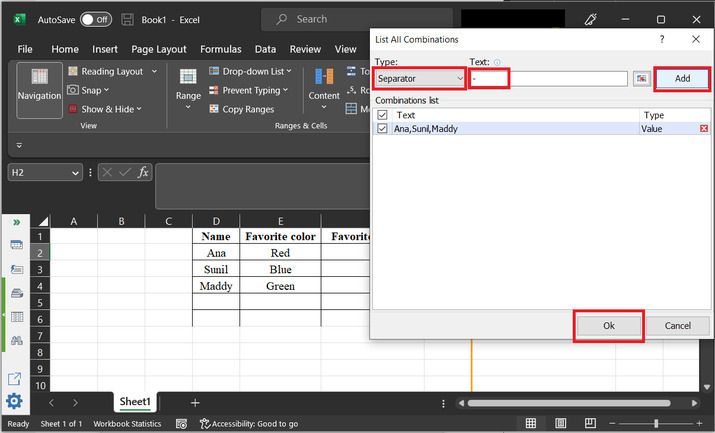### Step 6

Again, click on the box, button and select the data range from the E column. This will select the data as given below. Again, click on “OK” button.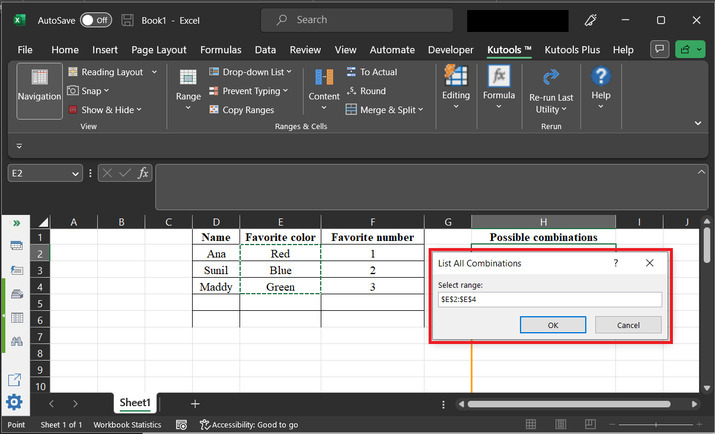### Step 7

Again, set a separator, use same steps as done before, and click on “Add”. Data will be appeared in the “List All Combination” dialog box.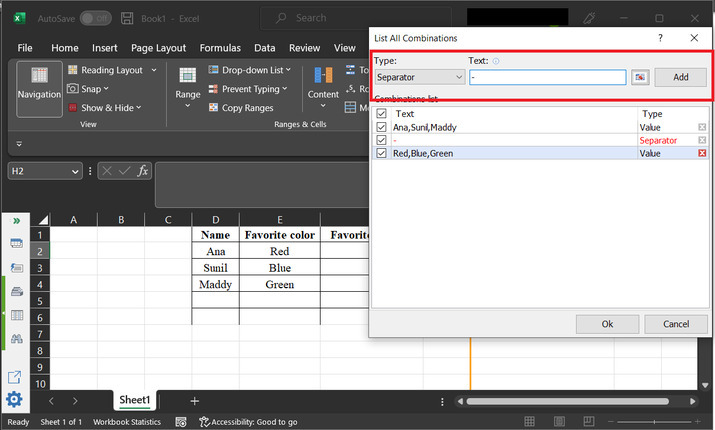### Step 8

Similarly, select data from the “F” column.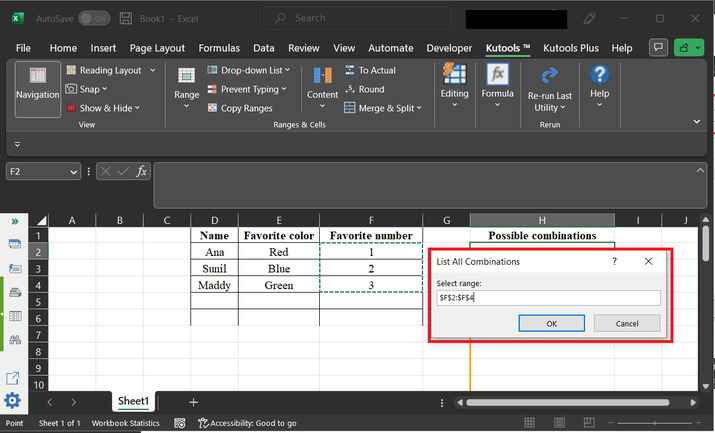### Step 9

Again, set a separator value with “-” sign. Finally, click on “Ok”.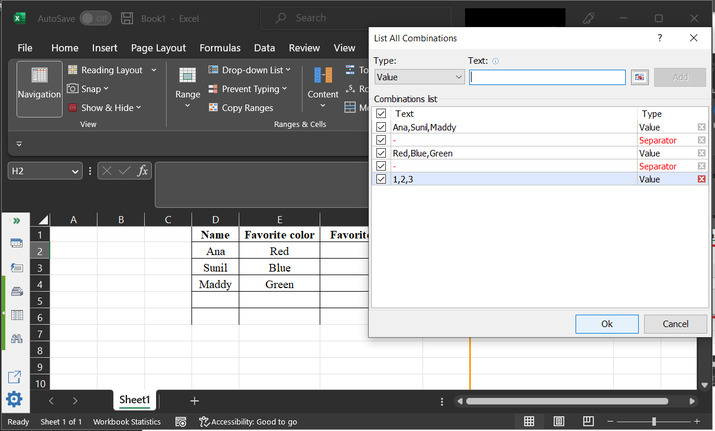### Step 10

This will close the dialog box. A new dialog box, for “List All Combinations” appear on console with an input option. In this input space, pass the column value, where user wants to enter the data.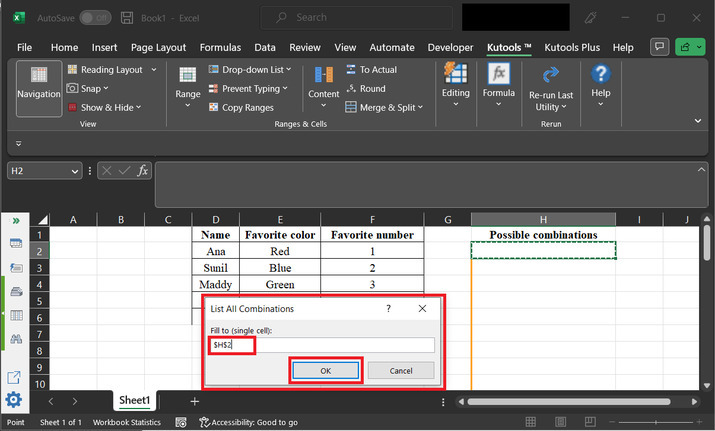### Step 11

Finally, obtained results are given below −## Conclusion

After learning all the three methods, user will be able to generate the combination of 3 or more columns efficiently, without any miss. All the three provided methods are simple and easy to use.

Updated on: 08-May-2023

2K+ Views